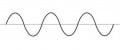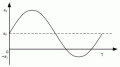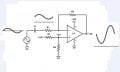What is DC Offset for?

Jean SP

Joined Feb 11, 2018
13
Hi, so i watched this video
about using arduino to turn on light with clap.
However i am more curious of how it really works, i dont get the part where it uses amplifier to increase the DC offset. What exactly
does the DC offset do.

PS: i understand that the amplifier amplify the signal so that there are more voltage steps to work with.

freak101

Joined Aug 7, 2017
37
This is our good old sin waveNow what is its area ? You can see that in a cycle, positive part is same as negative part. So its area is zero (integrate sin x in 0 to 2*pi).
Now this is another sine wave.Its area in 1 cycle is not zero. Thats because we have DC component in it.
DC offset is nothing but the average value !

Jean SP

Joined Feb 11, 2018
13
This is our good old sin wave
View attachment 145666
Now what is its area ? You can see that in a cycle, positive part is same as negative part. So its area is zero (integrate sin x in 0 to 2*pi).
Now this is another sine wave.
View attachment 145667
Its area in 1 cycle is not zero. Thats because we have DC component in it.
DC offset is nothing but the average value !
Thanks for replying. Does this mean a0 is the DC offset ? and why is it necessary to amplify the dc offset in the video. Appreciate your help!

GopherT

Joined Nov 23, 2012
7,983
Thanks for replying. Does this mean a0 is the DC offset ? and why is it necessary to amplify the dc offset in the video. Appreciate your help!

A pure ac signal is essentially balanced on each side of 0Volts.

If you want to manipulate the signal (amplify for example), you need to change the "frame of reference" of the signal so it is between the voltages of your power supply. If you are using a 9v battery for power, a DC offset offset of 4.5v may be reasonable. I say "may" because some amplifier circuits may be better with a much smaller offset. Does that help?

•Jean SP

Jean SP

Joined Feb 11, 2018
13
A pure ac signal is essentially balanced on each side of 0Volts.

If you want to manipulate the signal (amplify for example), you need to change the "frame of reference" of the signal so it is between the voltages of your power supply. If you are using a 9v battery for power, a DC offset offset of 4.5v may be reasonable. I say "may" because some amplifier circuits may be better with a much smaller offset. Does that help?
Thanks for replying ! I still don't quite get the idea of how the signal(Dc offset) is before amplify and after amplify. So the video uses an electric microphone which produce AC signal (sound) and DC signal (power microphone). and the DC signal is filtered using a capacitor. However because of the low voltage signal, it has to be amplify. So how the amplifier affect the DC Offset and how does the increase of DC offset be useful in this context. Thanks a lot!

ebp

Joined Feb 8, 2018
2,332
The gain of the circuit for the DC offset is one ("unity").
C1 blocks DC, so at DC the feedback around the amplifier is just R4, setting the non-inverting gain to one.

This is a very common circuit for amplifying a small AC signal using a "single ended" (unipolar) power supply.
Like you say, the signal from an electret mic already has a large DC component, but it is usually poorly defined, so it is AC coupled with a capacitor to the down-stream amplifier.

It is good practice to put a capacitor across R3 to filter any noise that might be present on the +5V supply, since that noise will be amplified. To do the job properly may require a substantial amount of capacitance (several microfarads), so a tantalum or aluminum electrolytic in parallel with a ceramic cap is commonly used. For just detecting a loud noise from the mic, it probably won't really be of practical benefit. If you are trying to digitize other audio, it should be used. If all you are doing is the clap detection, an NE5534 has far more performance and costs more than necessary.

MrChips

Joined Oct 2, 2009
19,160
Opamps are usually powered by one or two supplies.

In the classic opamp, there are two power supplies, one positive and the other negative. This is known as a bipolar power supply. The advantage of this is that the opamp is biased around 0V and it can amplify both positive and negative voltages (bipolar) to give bipolar output voltages.

Of course, the disadvantage is that you need two power supplies.
Hence comes the single supply opamp.

Can we power the opamp from a single supply?
Note that what we call 0V (or ground reference) in a circuit is arbitrary.
So what if we call the negative supply rail 0V reference instead?

That is what we do in a single supply opamp circuit. We have created a new 0V reference point. What was before our 0V reference point has now be shifted upwards to half the total supply voltage (which we will now call our pseudo ground). For our new circuit to work properly, everything that was previously at 0V reference has to be shifted upwards to our pseudo ground.

Hence if our single supply voltage is 5V, our pseudo ground is 2.5V.
That is what the DC shift is all about.

Hope this helps.

•anhnha

Jean SP

Joined Feb 11, 2018
13
Opamps are usually powered by one or two supplies.

In the classic opamp, there are two power supplies, one positive and the other negative. This is known as a bipolar power supply. The advantage of this is that the opamp is biased around 0V and it can amplify both positive and negative voltages (bipolar) to give bipolar output voltages.

Of course, the disadvantage is that you need two power supplies.
Hence comes the single supply opamp.

Can we power the opamp from a single supply?
Note that what we call 0V (or ground reference) in a circuit is arbitrary.
So what if we call the negative supply rail 0V reference instead?

That is what we do in a single supply opamp circuit. We have created a new 0V reference point. What was before our 0V reference point has now be shifted upwards to half the total supply voltage (which we will now call our pseudo ground). For our new circuit to work properly, everything that was previously at 0V reference has to be shifted upwards to our pseudo ground.

Hence if our single supply voltage is 5V, our pseudo ground is 2.5V.
That is what the DC shift is all about.

Hope this helps.
Hi ! Thanks for replying. Just to confirm my understanding , we make the dc offset to be 2.5v for the circuit to work properly ? And how does R2 in this case make the offset to be 2.5v ? Thank you !

Audioguru

Joined Dec 20, 2007
11,251
The circuit shown has errors:
1) The minimum allowed supply for an NE5534 is 6V but this circuit has only 5V.
2) The input voltage offset is +1.67V but some NE5534 opamps need at least 3V.
3) The output voltage offset is trying to be +3.33V but many NE5534 opamps outputs cannot go that high using a 5V supply.

If the input of an opamp is biased at half the supply voltage then the output of most modern opamps can swing equally up and down.

•Jean SP

Jean SP

Joined Feb 11, 2018
13
The circuit shown has errors:
1) The minimum allowed supply for an NE5534 is 6V but this circuit has only 5V.
2) The input voltage offset is +1.67V but some NE5534 opamps need at least 3V.
3) The output voltage offset is trying to be +3.33V but many NE5534 opamps outputs cannot go that high using a 5V supply.

If the input of an opamp is biased at half the supply voltage then the output of most modern opamps can swing equally up and down.
Thank you for replying! i have went to look for similar circuit and found this (ss attached below). Does this mean that by connecting a voltage divider at the non inverting input is to create DC offset? and why is it needed for that. From the video, it says that by connecting the non inverting input to a DC offset , it can amplify the AC signal without amplifying any DC voltage. i still don't quite understand how DC offset is for in this circuit. Appreciate all the help!

PS:

MrChips

Joined Oct 2, 2009
19,160
I guess my explanation in post #8 didn't help.Jean SP

Joined Feb 11, 2018
13

Jean SP

Joined Feb 11, 2018
13
I guess my explanation in post #8 didn't help.Appreciate your help !! But i still don't get the idea of DC offset for amplifier.

MrChips

Joined Oct 2, 2009
19,160
Yep! This is an inverting amplifier. but when does the DC offset comes in play.
Hang on! We're getting there one step at a time.

Jean SP

Joined Feb 11, 2018
13
Where can we get +6V?

We can get +6V by dividing +12V by 2 using a voltage divider where R3 = R4 as in the diagram below.

View attachment 145807

Now we have a little problem to deal with. Can you spot the problem?
Okay i understand the voltage divider part. but i don't see any problem with the figure. Can you explain :/ thank you!

MrChips

Joined Oct 2, 2009
19,160
Okay i understand the voltage divider part. but i don't see any problem with the figure. Can you explain :/ thank you!
The problem is the +6V offset on the bottom side of the sinewave generator. Usually, any signal generator is referenced to GND.

Consider what happens when we turn the amplitude of the sinewave output down to 0V amplitude. The DC voltage of the inverting input of the opamp is at +6V. The voltage at the non-inverting input is also at +6V because of the voltage divider. In other words, the two inputs are at the same voltage. In general, any opamp circuit with proper negative feedback resistor will always have the inverting and non-inverting inputs at the same voltage. Any voltage difference would be amplified by the opamp's open-loop gain and the output of the opamp would hit the supply voltage rail (either V+ or V-). In our example shown, the output is at +6V, regardless of the circuit gain -R2/R1.

In order to reference the signal generator back to 0V, we have to break the DC path. We do this by "AC coupling" the signal generator to the inverting input of the opamp using a capacitor.With the capacitor in place, the signal generator can be referenced to any voltage. The voltage at the inverting input is still +6V.

Why?

That is because the role of the feedback resistor and very high open-loop gain of the opamp means that the output of the opamp has to reach a voltage to force the difference voltage at the two inputs to zero.

Now, does this circuit resemble your microphone amplifier circuit?

•absf and Doros